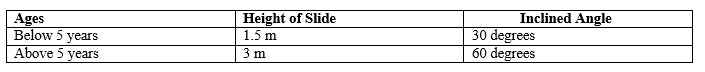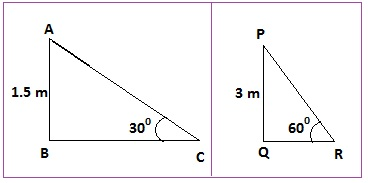# A contractor plans to install two slides for the children to play in a park. For the children below the age of 5 years, she prefers to have a slide whose top is at a height of 1.5 m, and is inclined at an angle of 30° to the ground, whereas for elder children, she wants to have a steep slide at a height of 3m, and inclined at an angle of 60° to the ground. What should be the length of the slide in each case?

As per contractor’s plan,Let, ABC is the slide inclined at 30° with length AC and PQR is the slide inclined at

60° with length PR.

To Find: AC and PR

In rt ∆ABC,

AB = 1.5m

AC = side = ?

P/H = AB/AC = sin30°

1.5/AC = 1/2

AC = 1/5 * 2

AC = 3m

In rt ∆PQR,

PQ = 3m

PR = side = ?

PH = PQ/PR = sin60°

3/PR = √3/2

√3 PR = 6

PR = 6/√3

6/√3 * √3/√3

= 2√3

= 2 * 1.73

= 3.46m